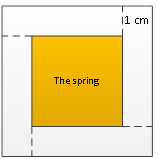### Sample Problem

A 1-meter wide road is around a square spring. The area of the road is 12 m2. What is the area of the spring?

m2#### Solution

We can divide the road into 4 rectangles of the same size, as shown.

The area of each rectangle is 12 ÷ 4 = 3 m2. Since we know the width to be 1 m, the length of each rectangle is 3 ÷ 1 = 3 m. The side length of the square is the rectangle length-the rectangle width which is 3 - 1 = 2 m. Therefore, the area of the spring is 2 × 2 = 4 m2.